## Filters

Sort by :
Clear All
Q

The transfer characterstic curve of a transistor, having input and output resistance  and  respectively, is shown in the figure. The Voltage and Power gain are respectively:• Option 1)

• Option 2)

• Option 3)

• Option 4)

=                                Option 1) Option 2) Option 3) Option 4)

The Figure represents a voltage regulator circuit using a Zener diode the break down voltage of Zener diode  is 6V and the load resistance is , The series resistance of the circut is . If the battery voltage varies from ,What are the minimum and maximum values of the current through Zener diode?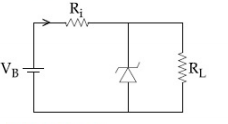• Option 1)

• Option 2)

• Option 3)

• Option 4)

Option 1)Option 2)Option 3)Option 4)

The truth table for the circuit given in the fig. is :• Option 1)

• Option 2)

• Option 3)

• Option 4)

NAND gate - NOT + AND gate - wherein A and B are input Y is out put     By putting values of the  cases, you can see that the option (1) is correct Option 1) Option 2) Option 3) Option 4)

An npn transistor operates as a common emmitter amplifier, with a power gain of

60 dB. The input circuit resistance is  and the output load resistance is

The common emitter cuttent gain  is :

• Option 1)

• Option 2)

• Option 3)

• Option 4)

Relation between α and β -   - wherein (current gain )     So, Option 1) Option 2) Option 3) Option 4)

The figure shows a DC voltage regulator circuit, with a Zener diode of breakdown voltage=6V. If the unregulated input voltage varies between 10 V to 16 V, then what is the maximum Zener current?• Option 1)

2.5 mA

• Option 2)

1.5 mA

• Option 3)

7.5 mA

• Option 4)

3.5 mA

Zener diode - It can operate continuously without being damaged in the region of reverse  biased - wherein 1) It acts as voltage regulator 2) In forward biasing it act as ordinary diode .     When the battery voltage is 16 V, the maximum current will be obtained Option 1) 2.5 mA Option 2) 1.5 mA Option 3) 7.5 mA Option 4) 3.5 mA

A common emitter amplifier circuit, built using an npn transistor, is shwon in the figure. Its dc current gain is 250,  and  what is the minimum base current for  to reach saturation?• Option 1)

• Option 2)

• Option 3)

• Option 4)

During saturation Current gain=  Option 1)Option 2)Option 3)Option 4)

The logic gate equivalent to the given logic circuit is :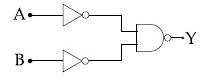• Option 1)

NAND

• Option 2)

OR

• Option 3)

NOR

• Option 4)

AND

Method - 1 TRUE  TABLE   Method - 2   Option 1) NAND Option 2) OR Option 3) NOR Option 4) AND

An NPN transistor is used in common emmitter configuration as an amplifire with  load resistance. Signal voltage of  is applied across the base-emitter. This produces a  change in the collector current and  change in the base current of the amplifier. The input resistance and voltage gain are :

• Option 1)

Drift along river flow

• Option 2)

• Option 3)

• Option 4)

Option 1)             Drift along river flow Option 2) Option 3)   Option 4)

The reverse breakdown voltage of a Zener diode is 5.6 V in the given circuit.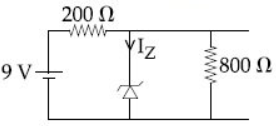The current through the Zener is :

• Option 1)

7 mA

• Option 2)

10 mA

• Option 3)

17 mA

• Option 4)

15 mA

So So, & So, Option 1)7 mAOption 2)10 mAOption 3)17 mAOption 4)15 mA

The circuit shown below contains two ideal diodes, each with a forward resistance of 50. If the battery voltage is 6V, the current through the 100 resistance(in Amperes) is:• Option 1)

0.036

• Option 2)

0.020

• Option 3)

0.030

• Option 4)

0.027

P -N junction as diode - It is a one way device. It offers a low resistance when forward biased and high resistance when reverse biased. - wherein R = 0, Forward  R  Reverse   In this situation   is in reverse bias so no current will pass through  &  Resistance. I=0.002 A  Option 1)  0.036Option 2)  0.020  Option 3)  0.030Option 4)  0.027

In the given circuit the current through Zener Diode is close to:• Option 1)

6.0 mA

• Option 2)

0.0 mA

• Option 3)

4.0 mA

• Option 4)

6.7 mA

Zener diode - It can operate continuously without being damaged in the region of reverse  biased - wherein 1) It acts as voltage regulator 2) In forward biasing it act as ordinary diode . Since voltage across zener must be less than 10 V , there for it will not work in break down region and its resistance is infinite and current through it is zero.    Option 1)6.0 mAOption 2)0.0 mAOption...

In the given figure, given that VBB supply can vary from 0 to 5.0 V,  , The minimum base current and the input voltage at which the transistor will go to saturation, will be, respectively :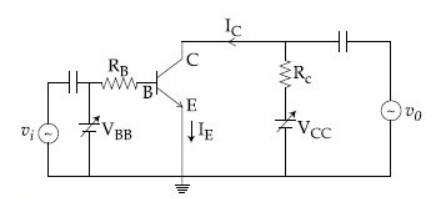• Option 1)

25  and 3.5 V

• Option 2)

25  and 2.8 V

• Option 3)

20  and 2.8 V

• Option 4)

20  and 3.5 V

Relation between emitter current ,Base current,collector current -   - wherein At saturation We known that So So     Option 1)25  and 3.5 VOption 2) 25  and 2.8 VOption 3)20  and 2.8 VOption 4)20  and 3.5 V

For the circuit shown below, the current through the Zener diode is: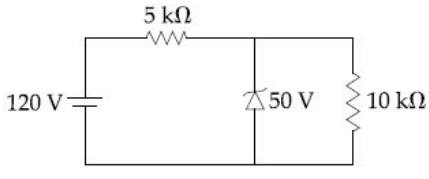• Option 1)

• Option 2)

• Option 3)

• Option 4)

Zero

Dynamic Resistance - - wherein Since slope of potential vs current graph is non uniform hence resistance keep changing .   Current through 10k, I2 = Current through 5k , Current through Zener = I - I2 = 14 - 5 = 9mAOption 1)Option 2)Option 3)Option 4)Zero

To get output '1' at R, for the given logic gate circuit the input values must be: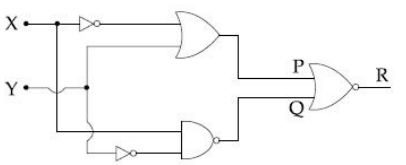• Option 1)

X=0, Y=1

• Option 2)

X=1, Y=1

• Option 3)

X=1, Y=0

• Option 4)

X=0, Y=0

D'morgan's Theorem - 1)     2)      3)     4)     - wherein A and B are input. we want and So For    Option 1)X=0, Y=1  Option 2)X=1, Y=1Option 3)X=1, Y=0Option 4)X=0, Y=0The output of the given logic circuit is :

• Option 1)• Option 2)• Option 3)• Option 4)D'morgan's Theorem - 1)     2)      3)     4)     - wherein A and B are input. or                                                                Option 1)Option 2)Option 3)Option 4)

Ge and Si diodes start conducting at 0.3V and 0.7V respectively. In the following figure if Ge diode connection are reversed, the value of V0 changes by:

(assume that the Ge diode has large breakdown voltage)• Option 1)

0.4 V

• Option 2)

0.6 V

• Option 3)

0.8 V

• Option 4)

0.2 V

P -N junction as diode - It is a one way device. It offers a low resistance when forward biased and high resistance when reverse biased. - wherein R = 0, Forward  R  Reverse     before reversing        (Since threshold voltage of  <   , so    conducts)     afer reversing   is reverse biased , so  conducts current  Option 1)0.4 VOption 2)0.6 VOption 3)0.8 VOption 4)0.2 V

Mobility of electrons in a semiconductor is defined as the ratio of their drift velocity to the applied electric field. If , for an n-type semiconductor, the density of electrons is 1019 m -3 and their mobility is 1.6 m2 / (V.s) then the resistivity of the semiconductor (since it is an n-type semiconductor contribution of holes is ignored ) is close to :

• Option 1)

• Option 2)

• Option 3)

• Option 4)

Electrical Conductivity (σ) -   - wherein For intrinsic semiconductors (no impurities), the number of electrons will be equal to the number of holes.  So        For N-type semiconductor electrons are majority carriers . Conductivity Resistivity = = 0.4 Option 1)Option 2)Option 3)Option 4)
Engineering
137 Views   |

A 2V battery is connected across AB as shown in the figure. The value of the current supplied by the battery when in one case battery’s positive terminal is connected to A and in other case when positive terminal of battery is connected to B will respectively be :• Option 1)

0.2 A and 0.1 A

• Option 2)

0.4 A and 0.2 A

• Option 3)

0.1 A and 0.2 A

• Option 4)

0.2 A and 0.4 A

As we have learned P -N junction as diode - It is a one way device. It offers a low resistance when forward biased and high resistance when reverse biased. - wherein R = 0, Forward  R  Reverse     In one case  NO current will pass through  I = 2/5 A = 0.4 A  In second case : no current will pass through  I = 2/10 = 0.2 A      Option 1) 0.2 A and 0.1 A   Option 2) 0.4 A and 0.2 A Option...
Engineering
313 Views   |

The circuit has two oppositely connect ideal diodes in parallel. What is the current following in the circuit?• Option 1)

1.33 A

• Option 2)

1.71 A

• Option 3)

2.00 A

• Option 4)

2.31 A.

As we have learnt,   P -N junction as diode - It is a one way device. It offers a low resistance when forward biased and high resistance when reverse biased. - wherein R = 0, Forward  R  Reverse     In the figure D, is reverse biased, hence no current will flow through D. Option 1)1.33 AOption 2)1.71 AOption 3)2.00 AOption 4)2.31 A.
Engineering
277 Views   |

In the following, which one of the diodes is reverse  biased?

• Option 1)• Option 2)• Option 3)• Option 4)As we have learnt,   P -N junction as diode - It is a one way device. It offers a low resistance when forward biased and high resistance when reverse biased. - wherein R = 0, Forward  R  Reverse    Correct option is 1 In all other figure, diode is forward biased.     Option 1)    Option 2) Option 3) Option 4)
Exams
Articles
Questions